# 7 Fast Calculation Tricks That Can Turn Your Child Into a Math Guru

Tips & tricks
9 months ago

Addition, subtraction, multiplication. These words instantly take us back to math class. And even if this class is just memory for us, there are still different things we need to count or solve in our daily lives. For our kids, they just need a way to get through math class quickly and easily. This whole process can become more engaging if we use a few tricks. And, no we’re not talking about the “dog ate my homework” trick.

## 1. Multiplication by 9, using your handsPlace your hands in front of you. Consider that each finger has its own number.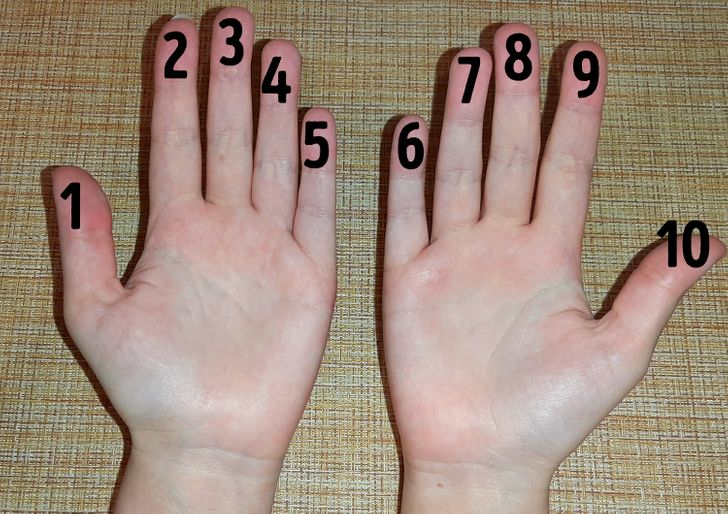As an example, let’s say you need to figure out 4×9. Find number 4 and bend your finger.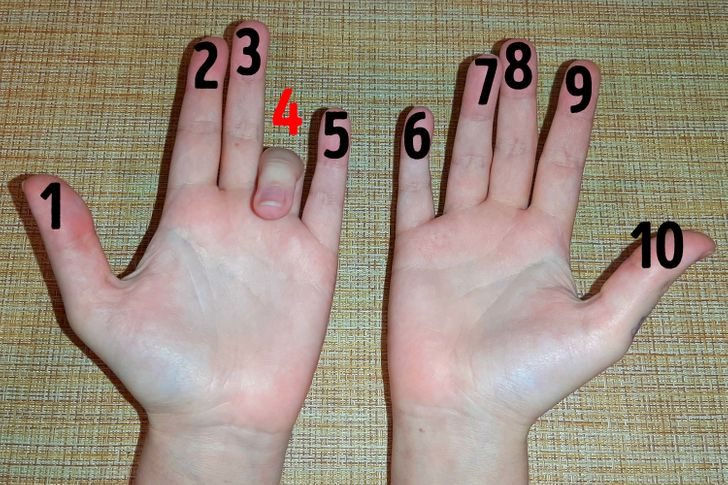Now count all the fingers to the left of the bent finger and to the right of the bent finger. On the left we have a 3, on the right we have a 6.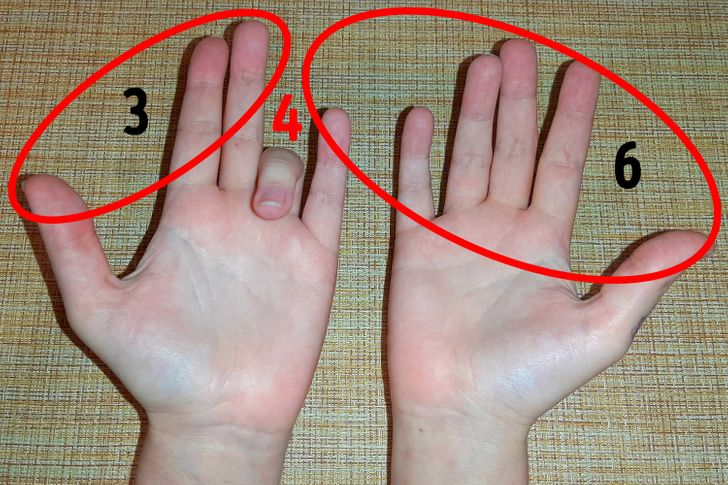Now put these numbers together. 36 is the correct answer.

## 2. Multiplication of 3-digit numbersAs an example, let’s say you need to solve 652×6. You can draw a table and write numbers out like in the picture above.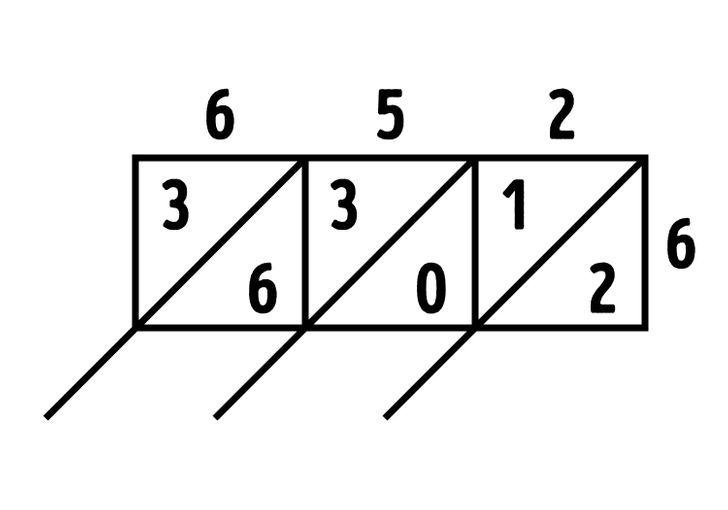Multiply each digit by 6 one by one and enter the result in the table.

6×6 = 36

5×6 = 30

2×6 = 12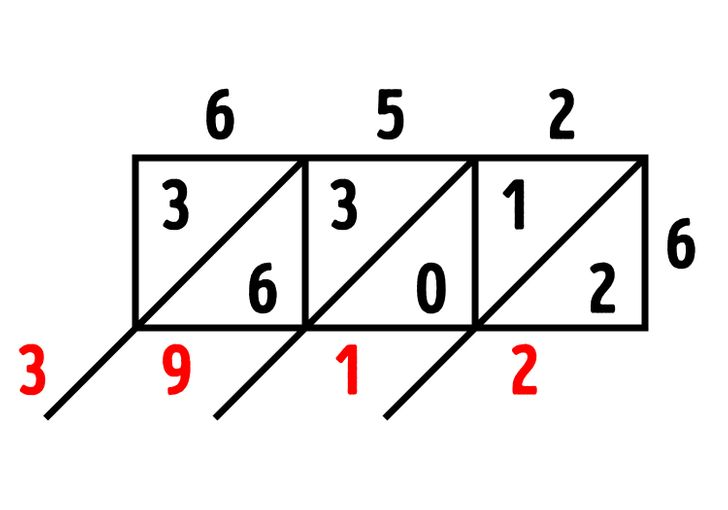Now add the numbers together, leaving the first and last ones unchanged. The resulting number will be the answer.

## 3. Multiplication of big numbers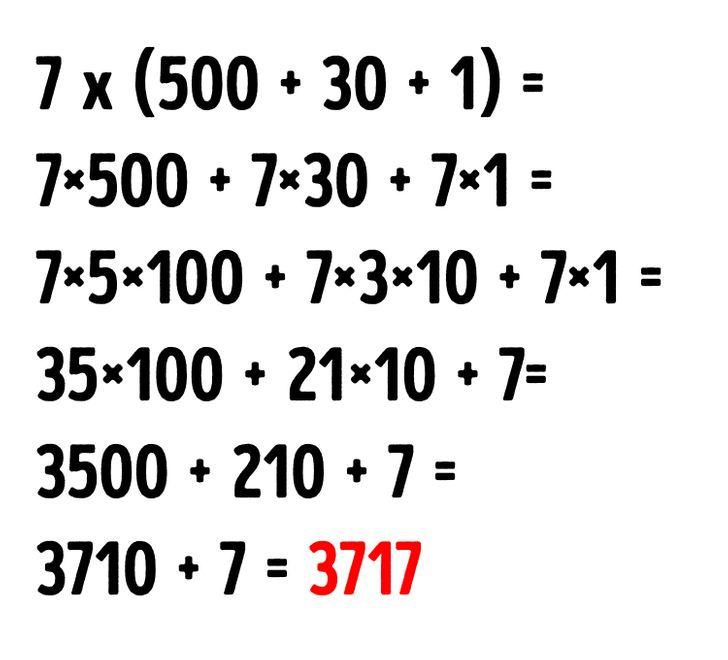As an example, let’s say you need to solve for 7×531. It can be easier to multiply, if you divide the big number up into rounded ones (10s, 100s). Try to make them as small as possible.

## 4. Multiplication by 12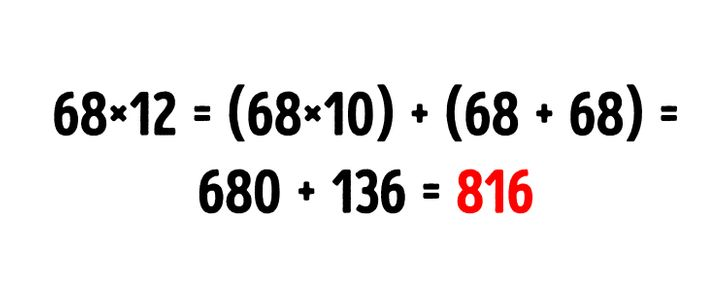## 5. The calculation of 15%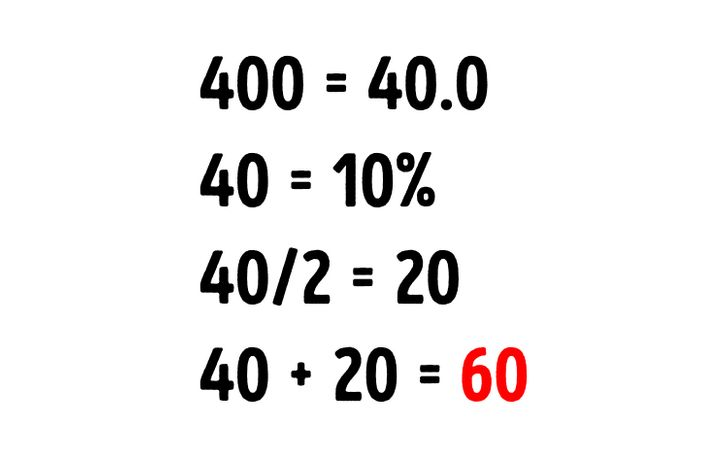If you’re trying to figure out 15% of a number, first you need to find 10%. Let’s take 400 as an example. Move the decimal point one character to the left. Divide this number by 2 and add the result to 40. The answer in this instance is that 60 is 15% of 400.

## 6. Addition of 3-digit numbers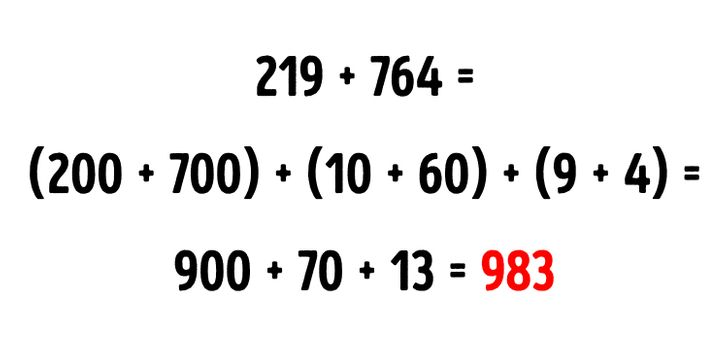3-digit numbers are easier to calculate when they are broken into parts. Add the 100s and 10s separately.

## 7. Multiplication by 9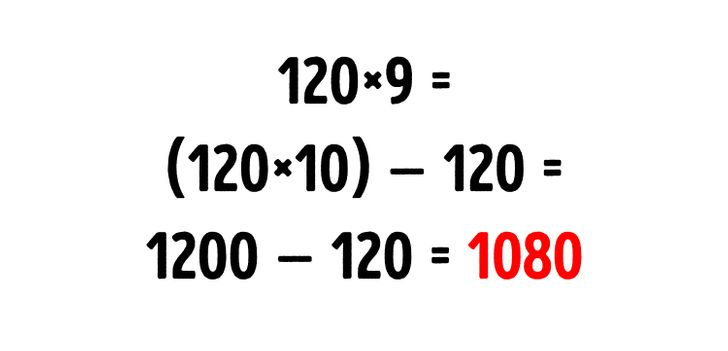You can multiply by 10 if you need to multiply by 9. Only don’t forget to subtract the original number from the result to get the correct answer.